Click to Chat

1800-1023-196

+91-120-4616500

CART 0

• 0

MY CART (5)

Use Coupon: CART20 and get 20% off on all online Study Material

ITEM
DETAILS
MRP
DISCOUNT
FINAL PRICE
Total Price: Rs.

There are no items in this cart.
Continue Shopping• Complete Physics Course - Class 11
• OFFERED PRICE: Rs. 2,968
• View Details

```Series R-L, R-C and L-C-R Circuits

Table of Content

Series R-L Circuit

Series R-C Circuit

Series L–C–R Circuit

Circuit Elements with AC

Related Resources

Circuits constitute a significant part of the Physics syllabus of IIT JEE. The R-L and R-C circuits are all the more important because the JEE often picks up questions form these topics. The series L-C-R circuit is also very crucial and is closely linked with the R-L circuit and the R-C series.

We shall now discuss the RC and RL circuits one by one followed by the L-C-R circuit.

Series R-L Circuit

Majority of coils that you see in the real world, whether they are solenoids, relays or any other similar component always exhibit certain amount of resistance because the copper wire has a resistive value. In such a case, the simple coil can then be considered as an Inductance in series with a resistance. This may be termed as the RL Series Circuit.
We have shown the RL Circuit in the figure given below. The circuit basically consists of an inductor of inductance L connected in series with a resistor of resistance R. This ‘R’ is the DC resistive value of the loops that is utilized in the preparation of the coil of the inductor.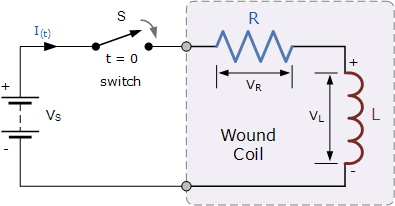As it is visible form the figure above, the circuit is attached to a battery and switch. The switch ‘S’ is assumed to be open till it is closed at time t = 0. It will then remain constantly closed thus producing a "step response" type voltage input. The current ‘i’ though flows through the circuit, but will not rise to its maximum value of Imax.

This restriction is basically due to the existence of the self-induced emf inside the inductor due to the growth of magnetic flux. After some time, the influence of the self- induced emf is nullified by the voltage source. The flow of current then becomes constant and the induced current and field are reduced to zero.

Watch this Video for more reference​

Series R-C Circuit

Potential difference across a capacitor in ac lags in phase by 90° with the current in the circuit. Suppose, in phasor diagram current is taken along positive x-direction. Then VR is also along positive x-direction but VC is along negative y-direction. So we can write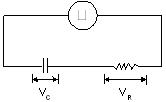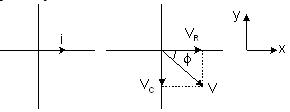V = VR – jVC = iR – j(iXC)
= iR – j (1/ωC)
= iZ
Here, impedence is given by
Z = R – j (1/ωC)
The modulus of impedance is,
| Z | =√R2+ (1/ωC)2
Also, the potential difference lags the current by an angle,
Φ = tan-1 | VC/VR| = tan-1 Xc /R = tan-1{(1/ωc)/ R}
Hence, Φ = tan -1(1/ωCR).
Let us now discuss the R-C circuit in detail:

The figure below shows a capacitor, (C) in series with a resistor, (R) forming a RC Charging Circuit connected across a DC battery supply via a mechanical switch. Once the switch is closed, with the help of the resistor, the capacitor gradually gets charged till the voltage across it reaches the supply voltage of the battery. The whole process of the charging of capacitor is depicted below.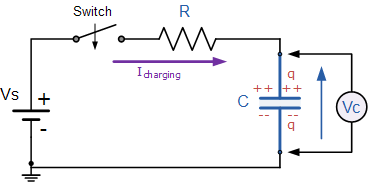Suppose that the capacitor (C) is fully discharged and the switch (S) is fully open. These are the initial conditions of the circuit when t =0, i=0 and q=0. The time commences when t=0 and it is then that the current begins to flow into the capacitor through the resistor. But the initial voltage (VC =0) of the capacitor is zero which forces the capacitor to appear as a short circuit and the maximum flow of current through the circuit is restricted by the resistor R. The current not flowing around the circuit is called the Charging Current and is calculated with the help of Ohms law.

Remarks:

Impedance: The total measure of opposition to electric current is called as impedance. It is in fact the vector sum of real resistance and imaginary resistance.

Impedance is denoted by Z and is managed just like resistances R in series circuits.

ZTotal = Z1 + Z2 + . . . Zn, so the series impedances add to form the total impedance. All the calculations involved in this should be performed in complex form and not in scalar form.

When resistors and capacitors are mixed together in circuits, the total impedance will have a phase angle somewhere between 0o and -90o.

The fundamental properties of Series AC circuits and series Dc circuits are the same. Current is uniform throughout the circuit, voltage drops add to form the total voltage, and impedances add to form the total impedance.

Series L–C–R Circuit

Potential difference across an inductor leads the current by 90° in phase while that across a capacitor, it lags in phase by 90°.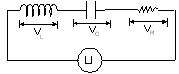Suppose in a phasor diagram current is taken along the positive x-direction. Then VR is along positive x-direction, Then VR is along positive x-direction, VL along positive y-direction and VC along negative y-direction.So, we can write, V = VR + jVL – jVC = iR + j(iXL) – j(iXC)

= iR + j [i (XL – XC)] = iZ

Here impedance is,

Z = R + j (XL – XC)

= R + j (ωL – 1/ωC)

The modulus of impedance is,

|Z| = √[R2 + (ωL – 1/ωC)2]

And the potential difference leads the current by an angle,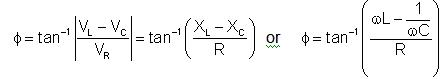We shall now work out some illustrations on how to calculate potential difference across an inductor in L-C-R.

Illustration: An alternating emf 200 virtual volts at 50 Hz is connected to a circuit of resistance 1Ω and inductance 0.01 H. What is the phase difference between the current and the emf in the circuit? Also find the virtual current in the circuit.

Solution: In case of an AC, the voltage leads the current in phase by an angle,
Φ = tan-1 (XL/R)
Here, XL = ωL = (2∏fL) = (2∏) (50) (0.01) = ∏Ω
and    R = 1Ω
∴Φ = tan-1 (∏) ≈ 72.3°
Further,
ims = Vms/ |Z| = Vms/√(R2+XL2)
Substituting the values we have,
ims = 200/√(1)2 + (π)2 = 60.67amp

Illustration: Find the voltage across the various elements, i.e., resistance, capacitance and inductance which are in series and having values 1000Ω, 1μF and 2 henry respectively. Given emf is, V = √100 sin 1000 t volt.

Solution: The rms value of voltage across the source,

Vms = 100√2/√2 = 100 voltsThe current will be same everywhere in the circuit, therefore

Potential Difference across resistor VR = irmsR = 0.0707 ∗1000 = 70.7 volt

Potential Difference across inductor VL = irmsXL = 0.0707 ∗1000 ∗ 2 = 141.4 volt and

Potential Difference across capacitor VC = irmsXC = 0.0707 ∗  = 70.7 volt

Note:
(i) The rms voltages do not add directly as

VR + VL + VC = 282.8 volt

Which is not the source voltage 100 volts. The reason is that these voltages are not in phase and can therefore be added by vector or by phasor algebra.

Circuit Elements with AC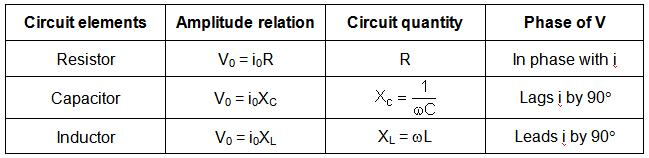It is imperative for the JEE aspirants to understand these circuits and their diagrams because the IIT JEE questions on induction and AC circuits are not very tough. The topic R-C and R-L circuits are also very important.

Related Resources

Look into the Previous Papers with Solutions to get a hint of the kinds of questions asked in the exam.

You can get the knowledge of Most Useful Books of Physics here.

```### Course Features

• 101 Video Lectures
• Revision Notes
• Previous Year Papers
• Mind Map
• Study Planner
• NCERT Solutions
• Discussion Forum
• Test paper with Video Solution